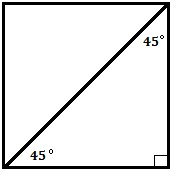Problems
329 - Simply Area II

Simply Area II

Time Limit: 1 sec

The Problem

Suppose you are standing in a Square room. There is a 45 degree angle line is drawn. Can you tell me what the Area of that room is?

Note: If the Diagonal of a Square is d then the Area of Square is d2 / 2.The Input

The input file contains a series of line. Each line contains an integer number length of draw line, L (L <= 9999) in cm. Input is terminated by EOF

The Output

For each line of input the output will show the Area of the room rounded to 2 decimal points in meter square.

Sample Input

7616

4893

Sample Output

2900.17 m^2

1197.07 m^2

Problem Setter: Samia Safa Ahmed